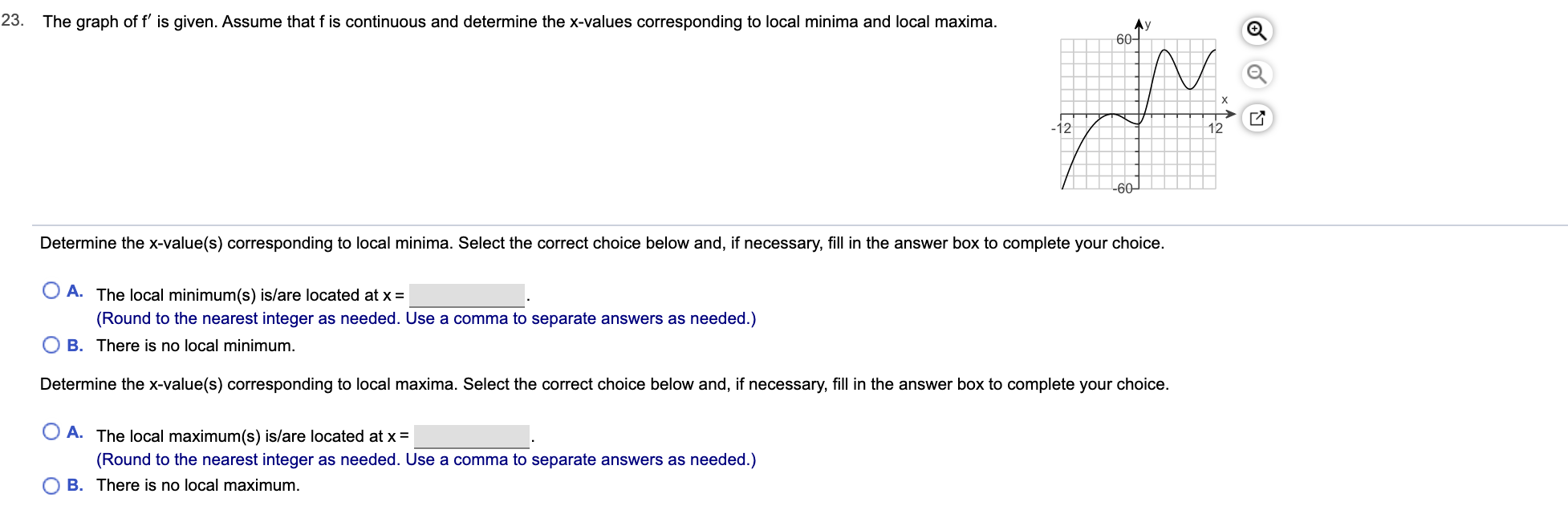# The graph of f' is given. Assume that f is continuous and determine the x-values corresponding to local minima and local maxima.23.Ay60-X-1212-60Determine the x-value(s) corresponding to local minima. Select the correct choice below and, if necessary, fill in the answer box to complete your choice.A. The local minimum(s) is/are located at x(Round to the nearest integer as needed. Use a comma to separate answers as needed.)B. There is no local minimum.Determine the x-value(s) corresponding to local maxima. Select the correct choice below and, if necessary, fill in the answer box to complete your choice.A. The local maximum(s) is/are located at x =(Round to the nearest integer as needed. Use a comma to separate answers as needed.)O B. There is no local maximum.

Question
24 views

Can you help with this problem step by step?help_outlineImage TranscriptioncloseThe graph of f' is given. Assume that f is continuous and determine the x-values corresponding to local minima and local maxima. 23. Ay 60- X -12 12 -60 Determine the x-value(s) corresponding to local minima. Select the correct choice below and, if necessary, fill in the answer box to complete your choice. A. The local minimum(s) is/are located at x (Round to the nearest integer as needed. Use a comma to separate answers as needed.) B. There is no local minimum. Determine the x-value(s) corresponding to local maxima. Select the correct choice below and, if necessary, fill in the answer box to complete your choice. A. The local maximum(s) is/are located at x = (Round to the nearest integer as needed. Use a comma to separate answers as needed.) O B. There is no local maximum. fullscreen
check_circle

Step 1

The problem is concerned with the critical points (maximum, minimum) of the given graph

Step 2

The local minima correspond to the points x=c such that f(x)>f(c) in some neighbourhood of c.(x not eq...

### Want to see the full answer?

See Solution

#### Want to see this answer and more?

Solutions are written by subject experts who are available 24/7. Questions are typically answered within 1 hour.*

See Solution
*Response times may vary by subject and question.
Tagged in

### Calculus